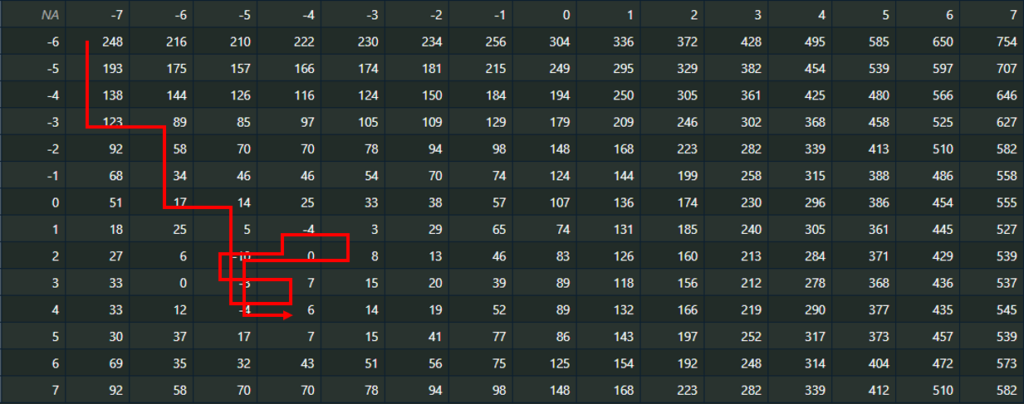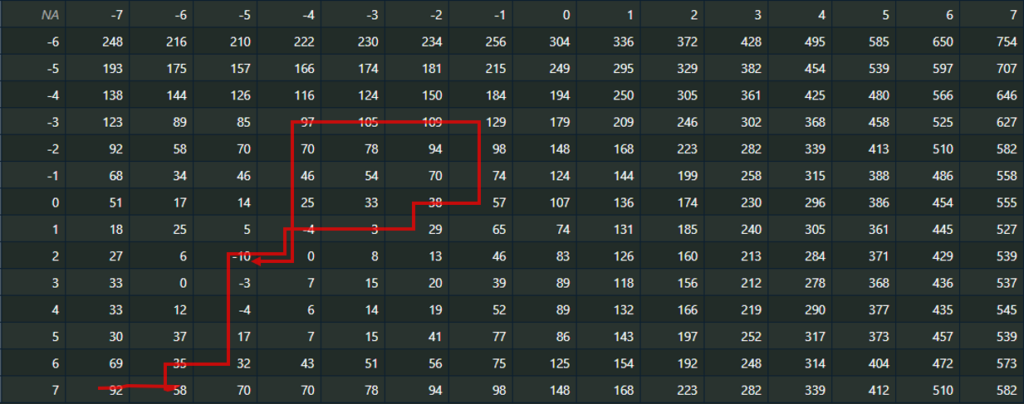# Tabu Search in R

Here I’ll show you a way, how to do Tabu Search with Steepest Descent with best improvement in R. Tabu Search is a local improvement heuristic used to improve existing solutions. Such heuristics are also called local search heuristics.

#### How does Local Search work?

Local search can be described in words as follows:

1. Take initial solution as a current solution
2. Calculate the neighborhood of the current solution applying an operation
3. Calculate objective function for some/all neighborhood-solutions
4. Based on a solution selection method, choose a better solution to be the next current solution or stop, if solution cannot be improved
5. Continue with step 2

Selection method can either be first improvement or best improvement. Among all solutions in the neighborhood, first improvement selects the first solution being better than the current solution, whereas best improvement needs to calculate the objective function for all candidates in the neighborhood and chooses the best one.

#### How does Tabu Search work?

What is added to the Local Search in order to get a Tabu Search is the fact, that

• the neighborhood is generated applying a set of different invertible operations or moves
• an additional constraint is introduced, preventing the application of some operations; this causes, that inverse operations to one of the previously performed n operations is forbidden
• the stop criterion is typically defined by reaching a maximum iteration limit
• as the final solution the best solution among all solutions visited will be returned

Compared to the Local Search, this local improvement heuristic has the advantage to explore a wider neighborhood and to improve the ability to escape from bad local optima. The Tabu Search does not, like Local Search does, stop, as soon as no better candidate can be found (greedy improvement), but rather accepts the current solution to become worse and thus have the chance to overcome bad local optima.

#### Showcase for Tabu Search in R

Let’s consider the following table to demonstrate Tabu Search with best improvement selection method to do minimization:

The objective here is to find the minimal value in the table applying Tabu Search, starting from a given initial solution with a tabu-list size of 1 move. Let’s say, we start with the initial solution at location x=-7, y=-6 which is 248. Then we have to do the following steps:

• check as neighborhood all numbers right/left or above/beneath the current solution. Here this would be: 216, 193
• Since we want to do best improvement selection, we have to check the objective function for all neighbors and pick the best solution among the allowed moves (since we have a tabu-list size of 1, only the last move may not be reversed in the next move)
• Since the values already are the objective values and we want to minimize, we just have to select the lowest value among all the neighbors
• Now we set x=-7, y=-5 to be the current solution with current minimal objective function value of 193
• check as neighborhood all numbers right/left or above/beneath the current solution. Here this would be: 248, 175, 138
• ….

We continue so long, until we reach the iteration limit. Additionally we need to keep track of the best solution found so far. If we continue like this, the solution might look as follows:Solution starting Tabu Search from (-7, -6) with Tabu-Size of 1 move

We can clearly see that the tabu-list size of 1 doesn’t really help the heuristic to explore a much larger neighborhood than local search would explore.

So let’s now check what solution we’s get, when we start from (-7, 7) with a tabu-list size of 3:Solution starting Tabu Search from (-7, 7) with Tabu-Size of 3 moves

Here we can see, that the heuristic escapes from the first local optimum identified quite good and does explore a wider additional part of the solution space. That’s what we intend the heuristic to do, when applying Tabu Search.

We now want to start to implement a solution in R (see here for the complete script file):

``````#' start a tabu search with steepest descent and a chessboard-neighborhood
#' within the given data-field applying best improvement strategy
#'
#' @param dat the data-field
#' @param x0 start x-coordinate in data-field where search is started
#' @param y0 start y-coordinate in data-field where search is started
#' @param maxit maximal number of iterations
#' @param minimizaation TRUE to minimize values, FALSE else
#' @param tabu_size size of memory to prevent opposite moves
#'
#' @return the best solution found
#' @export
tabu_search <- function(dat, x0, y0, maxit = 100, minimization = TRUE, tabu_size = 1) {
num_it <- 0
cur_cand <- list(x=x0, y=y0, val=get_mat_value(dat, x0, y0), op=0)
best_cand <- cur_cand
last_ops <- c()
while(num_it < maxit) {
# cur_val <- get_mat_value(dat, cur_x, cur_y)
# best_val <- cur_val
# calc neighborhood
nb <- neighborhood(dat, cur_cand\$x, cur_cand\$y)
next_cand <- NULL
# check every neighbor solution attainable f
for (i in 1:length(nb)) {
cand <- nb[[i]]
# check, if operation not in tabu list
if (!get_opposite_move(cand\$op) %in% tail(last_ops, tabu_size)) {
# move allowed
if (is.null(next_cand))
next_cand <- cand
# check if it's better than tha last 'next' candidate and update, if so
# then check if it's better than best candiate and update that, if so
if (minimization) {
if (cand\$val < next_cand\$val)
next_cand <- cand
if (cand\$val < best_cand\$val)
best_cand <- cand
} else {
if (cand\$val > next_cand\$val)
next_cand <- cand
if (cand\$val > best_cand\$val)
best_cand <- cand
}
}
}
cur_cand <- next_cand
last_ops <- tail(c(last_ops, cur_cand\$op), tabu_size)
cat("next val: ", cur_cand\$val, "\n")
num_it <- num_it + 1
}
return(best_cand)
}``````

In the variable best_cand we always keep track of the best candidate and initialize it with the current start-solution given, whereas in the cur_cand variable we keep track of the current solution candidate we’re currently examining the neighbors for.

Then you see two loops. The outer loop is a while loop, which is only terminated if the number iteration counter reaches the maximum iteration limit.

The second loop is a for loop which steps through all candidates of the neighborhood. Inside it you can find the check to prevent moves from being undone within a certain length of moves, calling the get_opposite_move – method:

``````#' calculate opposite move
#'
#' @param move one of c(1,2,3,4)
#'
#' @return the opposite move
#' @export
get_opposite_move <- function(move) {
opp_move <- c(3, 4, 1, 2)
return(opp_move[move])
}``````

To determine the neighborhood, we can do something like this:

``````#' calculate the neighborhood of the given coordinate
#'
#' @param dat field of data
#' @param x x-coord of current point
#' @param y y-coord of current point
#'
#' @return a list of lists containing the neighborhood
#' @export
neighborhood <- function(dat, x = -7, y = 7) {
x_id <- which(dat[1,] == x)
y_id <- which(dat[,1] == y)
cnt <- 1
nb <- list()
if (length(x_id) == 0 || length(y_id) == 0)
stop("didn't find location in matrix provided.")
for (op in 1:4) {
x_temp_id <- x_id; y_temp_id <- y_id
if (op == 1) {
x_temp_id <- x_temp_id + 1
} else if (op == 2) {
y_temp_id <- y_temp_id - 1
} else if (op == 3) {
x_temp_id <- x_temp_id - 1
} else {
y_temp_id <- y_temp_id + 1
}
if (x_temp_id > 1 && y_temp_id > 1 && x_temp_id <= dim(dat) &&
y_temp_id <= dim(dat)) {
nb[[cnt]] <- list(x=dat[1, x_temp_id], y=dat[y_temp_id, 1],
val=dat[y_temp_id, x_temp_id], op=op)
cnt <- cnt + 1
}
}
return(nb)
}``````

It’s probably not the simplest way to go, however it’s easy to read. We now need one more function to get the value from the position translated to the matrix-coordinates, based on row 1/ column 1:

``````#' return matrix value at position indicated by x, y if x and y were taken as
#' coordinates found in the first column and first row of the given matrix
#'
#' @param dat the matrix / data-field
#' @param x the x coordinate of the value to be returned
#' @param y the y coordinate of the value to be returned
#'
#' @return the value at coordinate x/y of the data-field
#' @export
get_mat_value <- function(dat, x, y) {
x_id <- which(dat[1,] == x)
y_id <- which(dat[,1] == y)
if (length(x_id) == 0 || length(y_id) == 0)
stop("didn't find location in matrix provided.")
return (dat[y_id, x_id])
}``````

Now we can find the minimum starting from the left top corner, calling:

``````dat <- matrix(c(NA, -7, -6, - 5, - 4, - 3, - 2, - 1, 0, 1, 2, 3, 4, 5, 6, 7, -6,248, 216, 210, 222, 230, 234, 256, 304, 336, 372, 428, 495, 585, 650, 754, -5, 193, 175, 157, 166, 174, 181, 215, 249, 295, 329, 382, 454, 539, 597, 707, -4, 138, 144, 126, 116, 124, 150, 184, 194, 250, 305, 361, 425, 480, 566, 646, -3, 123, 89, 85, 97, 105, 109, 129, 179, 209, 246, 302, 368, 458, 525, 627, -2, 92, 58, 70, 70, 78, 94, 98, 148, 168, 223, 282, 339, 413, 510, 582, -1, 68, 34, 46, 46, 54, 70, 74, 124, 144, 199, 258, 315, 388, 486, 558, 0, 51, 17, 14, 25, 33, 38, 57, 107, 136, 174, 230, 296, 386, 454, 555, 1, 18, 25, 5, -4,3, 29, 65, 74, 131, 185, 240, 305, 361, 445, 527, 2, 27, 6, -10, 0, 8, 13, 46, 83, 126, 160, 213, 284, 371, 429, 539, 3, 33, 0, -3, 7, 15, 20, 39, 89, 118, 156, 212, 278, 368, 436, 537, 4, 33, 12, -4, 6, 14, 19, 52, 89, 132, 166, 219, 290, 377, 435, 545, 5, 30, 37, 17, 7, 15, 41, 77, 86, 143, 197, 252, 317, 373, 457, 539, 6, 69, 35, 32, 43, 51, 56, 75, 125, 154, 192, 248, 314, 404, 472, 573, 7, 92, 58, 70, 70, 78, 94, 98, 148, 168, 223, 282, 339, 412, 510, 582), byrow=T, nrow=15)

# invoke tabu search heuristic
sol <- tabu_search(dat, x0 = -7, y0 = -6, tabu = 1, maxit = 25)
print(sol)
sol <- tabu_search(dat, x0 = -7, y0 = 7, tabu = 3, maxit = 25)
print(sol)
``````

The solution turns out to be:

``````\$x
 -5
\$y
 2
\$val
 -10
\$op
 4``````

for the first example or

``````\$x
 -5
\$y
 2
\$val
 -10
\$op
 2``````

for the second example. Both times the optimal solution is correctly identified.

Now we know that we have a local minimum, also being the global minimum here at (-5/2) with a value of -10. We can easily verify the result checking the table.

In both cases the optimal solution is not the current candidate where the heuristic stops searching but is found during its guided trip thourgh the solution space.

The whole R script of this demonstration can be downloaded here.

Categories: